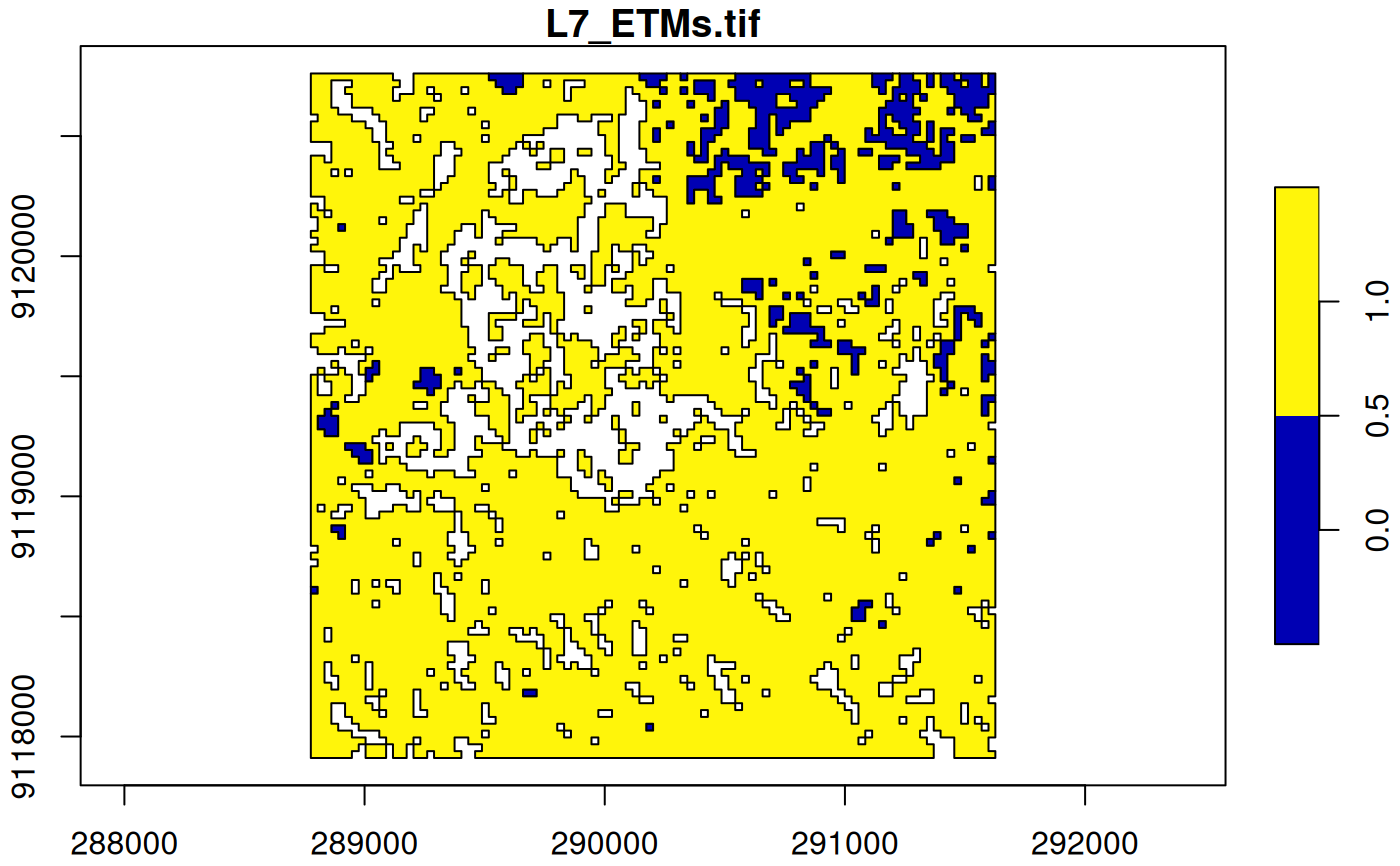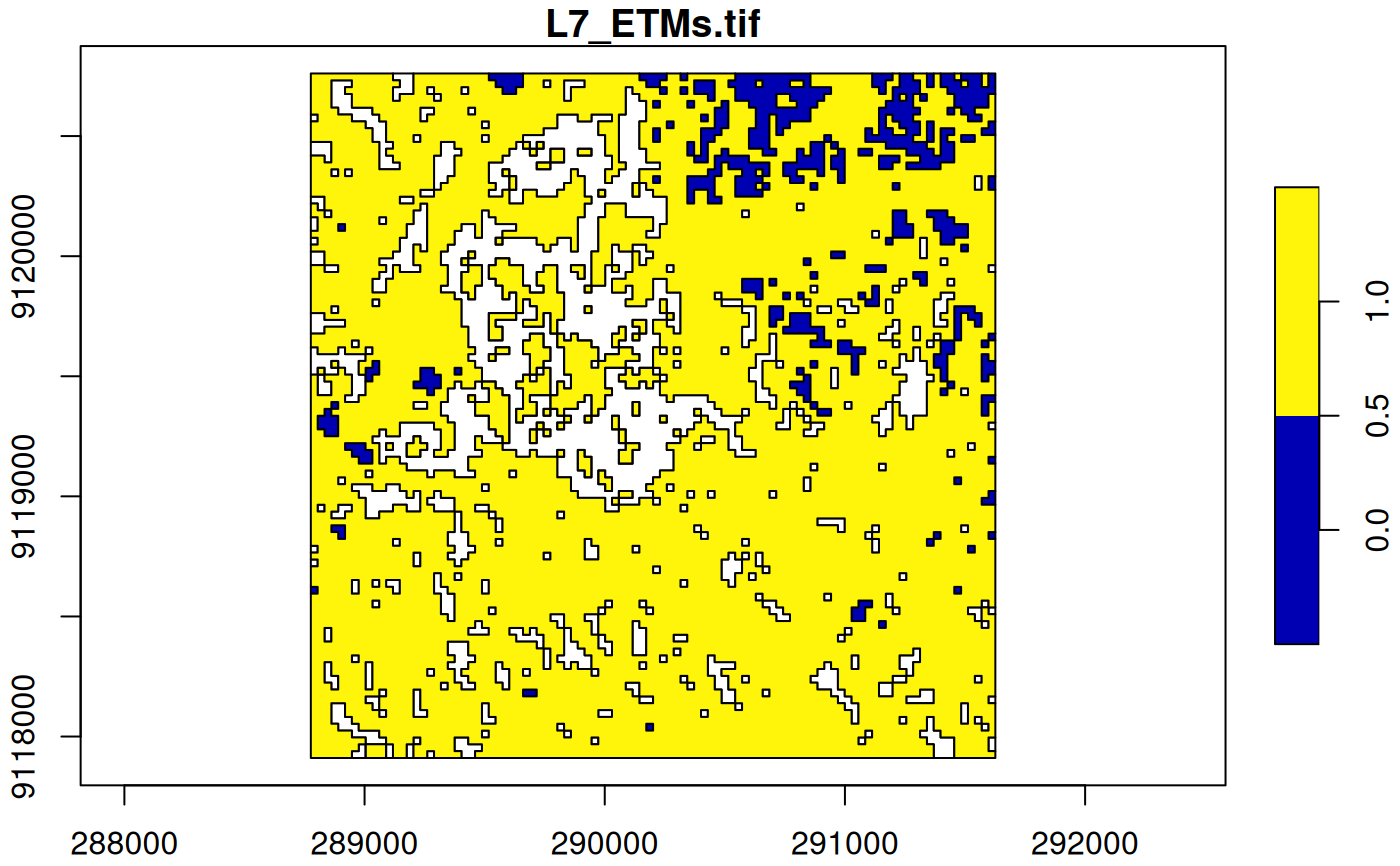Convert stars object into an sf object

# S3 method for stars
st_as_sfc(x, ..., as_points, which = seq_len(prod(dim(x)[1:2])))

# S3 method for stars
st_as_sf(
x,
...,
as_points = FALSE,
merge = FALSE,
na.rm = TRUE,
use_integer = is.logical(x[]) || is.integer(x[]),
long = FALSE,
connect8 = FALSE
)

# S3 method for stars_proxy
st_as_sf(x, ..., downsample = 0)

## Arguments

x

object of class stars

...

ignored

as_points

logical; should cells be converted to points or to polygons? See details.

which

linear index of cells to keep (this argument is not recommended to be used)

merge

logical; if TRUE, cells with identical values are merged (using GDAL_Polygonize or GDAL_FPolygonize); if FALSE, a polygon for each raster cell is returned; see details

na.rm

logical; should missing valued cells be removed, or also be converted to features?

use_integer

(relevant only if merge is TRUE): if TRUE, before polygonizing values are rounded to 32-bits signed integer values (GDALPolygonize), otherwise they are converted to 32-bit floating point values (GDALFPolygonize).

long

logical; if TRUE, return a long table form sf, with geometries and other dimensions recycled

connect8

logical; if TRUE, use 8 connectedness. Otherwise the 4 connectedness algorithm will be applied.

downsample

see st_as_stars

## Details

If merge is TRUE, only the first attribute is converted into an sf object. If na.rm is FALSE, areas with NA values are also written out as polygons. Note that the resulting polygons are typically invalid, and use st_make_valid to create valid polygons out of them.

## Examples

tif = system.file("tif/L7_ETMs.tif", package = "stars")
x = x[,1:100,1:100,6] # subset of a band with lower values in it
x[][x[] < 30] = NA # set lower values to NA
x[] = x[] < 100 # make the rest binary
x
#> stars object with 3 dimensions and 1 attribute
#> attribute(s):
#>  L7_ETMs.tif
#>  Mode :logical
#>  FALSE:525
#>  TRUE :7665
#>  NA's :1810
#> dimension(s):
#>      from  to  offset delta                     refsys point x/y
#> x       1 100  288776  28.5 SIRGAS 2000 / UTM zone 25S FALSE [x]
#> y       1 100 9120761 -28.5 SIRGAS 2000 / UTM zone 25S FALSE [y]
#> band    6   6      NA    NA                         NA    NA
(p = st_as_sf(x)) # removes NA areas
#> Simple feature collection with 8190 features and 1 field
#> Geometry type: POLYGON
#> Dimension:     XY
#> Bounding box:  xmin: 288776.3 ymin: 9117911 xmax: 291626.3 ymax: 9120761
#> Projected CRS: SIRGAS 2000 / UTM zone 25S
#> First 10 features:
#>      V1                       geometry
#> 1  TRUE POLYGON ((288776.3 9120761,...
#> 2  TRUE POLYGON ((288804.8 9120761,...
#> 3  TRUE POLYGON ((288833.3 9120761,...
#> 4  TRUE POLYGON ((288861.8 9120761,...
#> 5  TRUE POLYGON ((288890.3 9120761,...
#> 6  TRUE POLYGON ((288918.8 9120761,...
#> 7  TRUE POLYGON ((288947.3 9120761,...
#> 8  TRUE POLYGON ((288975.8 9120761,...
#> 9  TRUE POLYGON ((289004.3 9120761,...
#> 10 TRUE POLYGON ((289032.8 9120761,...
(p = st_as_sf(x[,,,1], merge = TRUE)) # glues polygons together
#> Simple feature collection with 155 features and 1 field
#> Geometry type: POLYGON
#> Dimension:     XY
#> Bounding box:  xmin: 288776.3 ymin: 9117911 xmax: 291626.3 ymax: 9120761
#> Projected CRS: SIRGAS 2000 / UTM zone 25S
#> First 10 features:
#>    L7_ETMs.tif                       geometry
#> 1            0 POLYGON ((289517.3 9120761,...
#> 2            0 POLYGON ((290144.3 9120761,...
#> 3            0 POLYGON ((290315.3 9120761,...
#> 4            0 POLYGON ((291113.3 9120761,...
#> 5            0 POLYGON ((291341.3 9120761,...
#> 6            1 POLYGON ((291455.3 9120761,...
#> 7            1 POLYGON ((291569.3 9120761,...
#> 8            0 POLYGON ((290372.3 9120732,...
#> 9            1 POLYGON ((290628.8 9120732,...
#> 10           1 POLYGON ((290771.3 9120732,...
all(st_is_valid(p)) # not all valid, see details
#>  TRUE
plot(p, axes = TRUE)(p = st_as_sf(x, na.rm = FALSE, merge = TRUE)) # includes polygons with NA values
#> Simple feature collection with 380 features and 1 field
#> Geometry type: POLYGON
#> Dimension:     XY
#> Bounding box:  xmin: 288776.3 ymin: 9117911 xmax: 291626.3 ymax: 9120761
#> Projected CRS: SIRGAS 2000 / UTM zone 25S
#> First 10 features:
#>    L7_ETMs.tif                       geometry
#> 1            0 POLYGON ((289517.3 9120761,...
#> 2            0 POLYGON ((290144.3 9120761,...
#> 3            0 POLYGON ((290315.3 9120761,...
#> 4            0 POLYGON ((291113.3 9120761,...
#> 5            0 POLYGON ((291341.3 9120761,...
#> 6            1 POLYGON ((291455.3 9120761,...
#> 7            1 POLYGON ((291569.3 9120761,...
#> 8           NA POLYGON ((289745.3 9120732,...
#> 9           NA POLYGON ((289830.8 9120732,...
#> 10           0 POLYGON ((290372.3 9120732,...
plot(p, axes = TRUE)